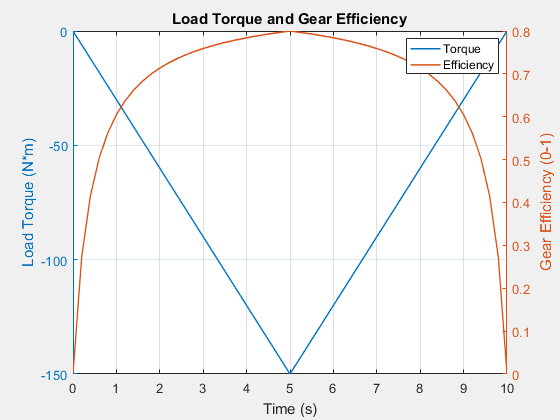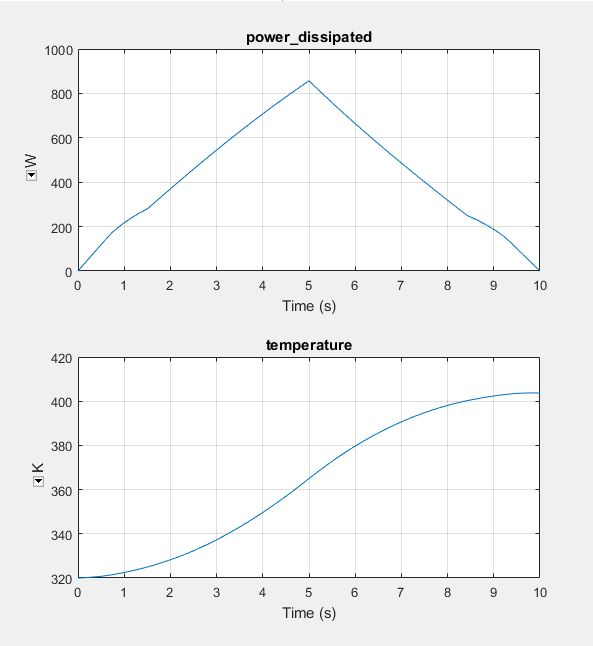## Model Thermal Losses in Driveline Components

Thermal modeling provides data that helps you to design efficiency and thermal protection into your system. Certain blocks in the Simscape™ Driveline™ Brakes & Detents, Clutches, and Gears libraries have thermal variants that allow you to determine how heat generation affects the efficiency and temperature of driveline components. For example, the Simple Gear block, which models a gear of base and follower wheels, has a thermal variant that can simulate the heat generated by meshing losses. Selecting a thermal variant for a block adds a thermal port to the block and enables the associated thermal-modeling parameters.

### Thermal Ports

Thermal ports are physical conserving ports in the Simscape thermal domain. You can model thermal effects like heat exchange and insulation by connecting blocks, from other Simscape products, that use the thermal domain to the thermal ports on Simscape Driveline thermal variants.

Thermal ports are associated with temperature and heat flow which are the Across and Through variables of the Simscape thermal domain. To measure thermal variables, you can use one or both of these methods:

1. Log simulation data using a Simscape logging node. View the data using the `sscexplore` function.

2. Add a sensor from the Simscape > Foundation Library > Thermal > Thermal Sensors library to your model. To measure temperature, use a parallel-connected Ideal Temperature Sensor block. To measure heat flow, use a series-connected Ideal Heat Flow Sensor block.

There are several advantages to using data logging for desktop simulation. Data logging is less computationally costly than using a gauge block and it allows you to:

• View post-simulation results easily using the Simscape Results Explorer.

• Output data easily to the MATLAB® Workspace for post-processing analysis.

However, if you use only data logging to measure a variable, you cannot output a feedback signal for that variable to a control system during simulation as you can when you use only a sensor to measure the variable. Also, because data logging is not supported for code generation, you cannot use Simscape data logging when you perform real-time simulation on target hardware.

### Thermal-Modeling Parameters

Thermal-modeling parameters are device-specific characteristics that determine how thermal dynamics affect device temperature and performance during simulation.

For some blocks, the default variant includes requisite parameters for simulating thermal dynamics. For such blocks, parameter dimensions change when you select a thermal variant. For example, to parameterize meshing losses based on a constant efficiency friction model for the default variant of the Simple Gear block, you specify the Efficiency parameter using a scalar value. If you select a thermal variant for the Simple Gear block, you must use a vector quantity to specify the Efficiency parameter.

Selecting a thermal variant enables additional thermal-modeling parameters. For example, selecting the thermal variant of the Simple Gear block enables the Temperature parameter. To determine the extent of thermal losses, the block performs a table lookup based on the values that specified for the Efficiency and Temperature parameters.

### Model Thermal Losses for a Simple Gear

This example shows how to enable a thermal port, parameterize a thermal variant, and analyze the results of a simulation that models thermal losses.

#### Measure Load-Dependent Efficiency

1. Open the model. At the MATLAB command prompt, enter

`sdl_gear_efficiency`

2. Examine the parameters for the Gear block.

For Meshing Losses, the Friction model is set to ```Load-dependent efficiency```. The Nominal output torque is `150 N*m` and the Efficiency at nominal output torque is `0.8`.

3. To simulate the model and plot gearbox efficiency, in the model window, click Plot efficiency.The efficiency at the nominal point exactly matches the parameter values in the block. However, the efficiency is dependent only on the torque. Temperature does not factor into the efficiency calculation.

#### Use a Thermal Variant for the Gear Block

To include temperature as a factor in the efficiency calculation, select a thermal variant for the gear block.

1. Right-click the Gear block and, from the context menu, select Simscape > Modeling option. Select Show thermal port.

2. Parameterize the thermal variant. If the block property inspector is open, close and then open it to make the thermal parameters visible. For the Meshing Losses parameters:

1. Set Friction Model to ```Temperature and load-dependent efficiency```.

2. For Temperature, specify ```[ 280 400 500 ]```.

3. For Efficiency matrix, specify ```[ 0.65 0.65 0.7; 0.7 0.7 0.75; 0.75 0.75 0.8 ]```.

3. For the Thermal Port > Initial Temperature parameter, specify 320.

#### Add Thermal Library Blocks

To model heat transfer, add blocks from the Simscape Foundation Thermal library.

1. Add a block that represents heat flow between the gear and the environment. Open the Simulink® Library Browser. From the Simscape > Foundation Library > Thermal > Thermal Elements library, add a Conductive Heat Transfer block to the model.

2. Add a block that represents a thermal reference point. Also from the Thermal Elements library, add a Thermal Reference block to the model.

3. Add blocks for modeling the ambient temperature as a constant, ideal source of thermal energy.

• From the Simscape > Foundation Library > Thermal > Thermal Sources library, add an Controlled Temperature Source block.

• From the Simscape > Foundation Library > Physical Signals > Sources library, add a PS Constant block. Specify a value of `320` for the PS Constant block.

4. Arrange and connect the blocks as shown in the figure.#### Measure Temperature and Load-Dependent Efficiency

Evaluate efficiency as a function of both the load torque and the gear temperature.

1. Simulate the model.

2. To plot gearbox efficiency, in the model window, click Plot efficiency. Zoom out for a better view of the efficiency curve.The efficiency peaks at 8.5 seconds when the magnitude of the load torque is approximately 33% of its maximal value. The efficiency is no longer dependent only on the load torque.

3. To view the data for the dissipated power and for gear temperature:

1. In the model window, click Explore simulation results.

2. In the node tree window, expand the Gear > simple_gear_model nodes.

3. CTRL + click the power_dissipated and temperature nodes.As the magnitudes of the torque load and temperature increase during the first half of the simulation, so does the amount of power that is dissipated. The amount of power that is dissipated decreases in the second half of the simulation due to the decrease in the torque load. However, the temperature of the gear continues to rise, as does the efficiency due to the vector specified for the Temperature parameter. The efficiency depends on both the load torque and the gear temperature.